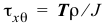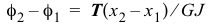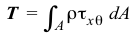# 3.2 – THEORY OF TORSION OF CIRCULAR SHAFTS

 In the questions below, select the correct answer. NameEmail1) Torsional shear strain varies linearly across a homogeneous cross section. True False2) Torsional shear strain is a maximum at the outermost radius for a homogeneous and a nonhomogeneous cross section. True False3) Torsional shear stress is a maximum at the outermost radius for a homogeneous and a nonhomogeneous cross section. True False4) The formulacan be used to find the shear stress on a cross section of a tapered shaft. True False5) The formulacan be used to find the relative rotation of a segment of a tapered shaft. True False6) The formulacan be used to find the shear stress on a cross section of a shaft subjected to distributed torques. True False7) The formulacan be used to find the relative rotation of a segment of a shaft subjected to distributed torques. True False8) The equationcannot be used for nonlinear materials. True False9) The equationcan be used for a nonhomogeneous cross section. True False10) Internal torques jump by the value of the concentrated external torque at a section. True FalseDo you have any comments or suggestions? Please email them to author@madhuvable.org